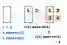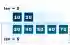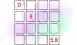# Python 2D List Examples

Create a list of lists, or a 2D list. Append empty lists to a list and add elements.

2D list. On a flat stone surface, we play a game of marbles. There is red, aquamarine, indigo. No marble is on top of another. The game is limited to 2 dimensions—just X and Y positions.Python list notes. In a list we can place other lists: this creates a 2D representation of objects (like glass marbles). We can access elements on each list with an index.List, strings

First example. We create an empty list and add empty lists to it with append(). We can construct any rectangular (or jagged) list this way. In this example we build a 2 by 2 list.
Step 1: We first create an empty list with the empty square brackets. Then we call the append method and add 2 empty lists.
Step 2: We access each sub-list and call append on it. This appends to the inner lists.
Step 3: We display the element at indexes 0, 0 and this value is 1. With the print() method we can display the entire list contents.
Step 4: This loop can iterate rows and columns in the 2D list. Nested for-loops loop over rows and columns.Python program that creates and adds to 2D list # Step 1: create a list. # ... Append empty lists in first two indexes. elements = [] elements.append([]) elements.append([]) # Step 2: add elements to empty lists. elements.append(1) elements.append(2) elements.append(3) elements.append(4) # Step 3: display top-left element. # ... Display entire list. print(elements) print(elements) # Step 4: loop over rows. for row in elements: # Loop over columns. for column in row: print(column, end="") print(end="\n") Output 1 [[1, 2], [3, 4]] 12 34

Jagged lists. The term "jagged" implies that sub lists have uneven lengths. Here we create a list of 2 lists—one of length 2, the other of length 5. We display their lengths.
Info: This is a list of lists, not exactly a 2D list. It does not need to be rectangular—some lists can be longer than others.
Tip: All the methods on list, including "len" and "in," work on nested lists. But they consider only one sub-list at a time.
Len
Caution: For performance, nested lists are not optimal. An array is faster than a list.
ArrayPython program that uses jagged lists # A jagged list. values = [[10, 20], [30, 40, 50, 60, 70]] for value in values: # Print each row's length and its elements. print(len(value), value) Output 2 [10, 20] 5 [30, 40, 50, 60, 70]

Flattened. It is possible to index into a 1D array as though it is two-dimensional. We add the first coordinate, "x," and then multiply the second "y" coordinate by the length.
Index: The multiplication of the coordinates returns a single integer for a 2D point.
Here: We define get_element and set_element methods. We compute indexes based on an "x" and "y" coordinate pair.Python program that uses flattened list def get_element(elements, x, y): return elements[x + (y * 4)] def set_element(elements, x, y, value): elements[x + (y * 4)] = value # Create a list of 16 elements. elements = [] for i in range(0, 16): elements.append(0) i = 0 for x in range(0, 4): for y in range(0, 4): # Set each element in the flattened list. set_element(elements, x, y, i) i += 1 # Print some elements. print(get_element(elements, 0, 0)) print(get_element(elements, 1, 1)) print(get_element(elements, 3, 3)) Output 0 5 15

Benchmark, nested list. Suppose it is simple to change a nested list to a flattened, 1D list. This will likely result in a performance increase.
Version 1: This version of the code accesses an element in a nested list—so it accesses 2 elements total.
Version 2: This code accesses the flattened list, using an expression to compute the correct index.
Result: Accessing the element at position coordinates 1, 2 is slightly faster in the flattened list.
Python program that times nested, flattened list import time # Nested, 3x2. nested_list = [] nested_list.append([10, 20, 30]) nested_list.append([40, 50, 60]) # Flattened, 3x2. flat_list = [10, 20, 30, 40, 50, 60] # Test the two lists. print(nested_list) print(flat_list[1 + (2 * 2)]) print(time.time()) # Version 1: access element in nested list. for i in range(0, 100000000): if nested_list != 60: print(False) print(time.time()) # Version 2: access element in flattened list. for i in range(0, 100000000): if flat_list[1 + (2 * 2)] != 60: print(False) print(time.time()) Output 60 60 1420077374.795 1420077375.334: Nested list, 0.539 s 1420077375.794: Flat list, 0.460 s [PyPy]

2D array. Python supports a special "array" from the array module. An integer array is more compact in memory than an integer list. We can create a flattened 2D array.2D Array

A review. Sometimes a 2D list is helpful in programs. For a simple or small coordinate system or grid, nested lists can be useful. They are flexible. The syntax is clear.

Larger data sizes. For larger or more complex requirements, this approach is not ideal. Another data structure, like an array, is a better choice at this scale.

Home
© 2007-2020 Sam Allen. Send bug reports to info@dotnetperls.com.